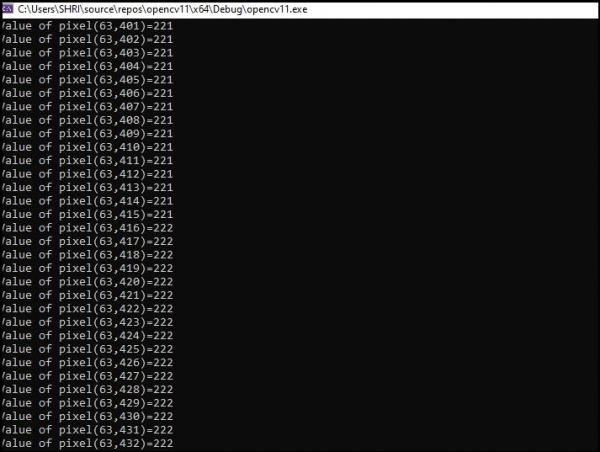# How to read the pixel value of a single channel image in OpenCV using C++?

Digital images are made of pixels. Using OpenCV, it is easy to read the value of pixels. However, if we want to get the pixel values, we have to handle a single channel separately.

Here we are loading an image in the matrix named 'cimage', and then it converts the image using 'cvtColor(cimage, img, COLOR_BGR2GRAY); ' and store it in the matrix named 'img'.

The following program read the pixel value of an image and shows the values in console window.

## Example

#include<iostream>
#include<opencv2/highgui/highgui.hpp>
#include<opencv2/opencv.hpp>
using namespace std;
using namespace cv;
int main() {
int x;//Declaring an integer variable to hold values of pixels//
Mat img;//Declaring an empty matrix to store converted image//
cvtColor(cimage, img, COLOR_BGR2GRAY);//Converting loaded image to grayscale image//
for (int i = 0; i < img.rows; i++)//loop for rows// {
for (int j = 0; j < img.cols; j++)//loop for columns// {
x = (int)img.at<uchar>(i, j);//storing value of (i,j) pixel in variable//
cout << "Value of pixel" << "(" << i << "," << j << ")" << "=" << x << endl;//showing the values in console window//
}
}
imshow("Show", img);//showing the image//
waitKey();//wait for keystroke from keyboard//
return 0;
}

## Output